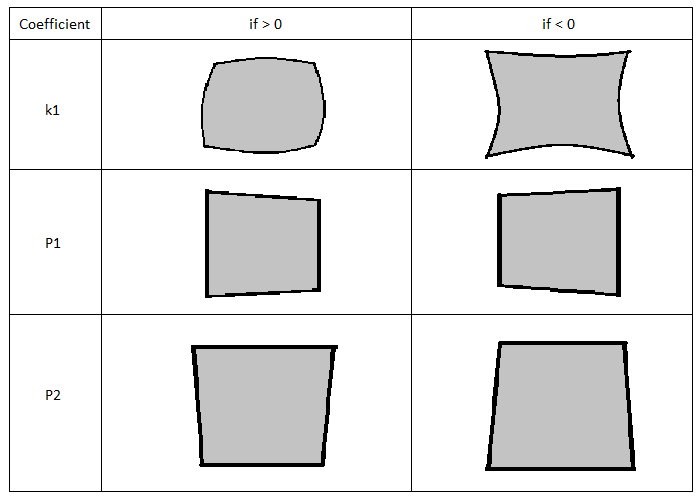## Camera-lens calibration

The coordinates on the sensor are expressed in a base of vectors called (u,v).

The distortion of a camera-lens system can be expressed by an operator that transforms the coordinates with distortion (u,v) into coordinates without distortion (u’,v’) or vice versa.

Therefore as a general rule we can write:

((u'),(v'))=A((u),(v))

With A, a generic operator.

Classic modelling requires A to be a function:

((u'),(v'))=1/(1+kr^2)((u),(v))

With

r^2=x^2+y^2

The factor can be developed in series to divide the contributions of the different degrees of r.

To also take spurious effects into account, the equation can be redefined as:

((u'),(v'))=((u(1+k_1r^2+k_2r^4+...)+P_1(r^2+2u^2)+2P_2uv+...),(v(1+k_1r^2+k_2r^4+...)+P_2(r^2+2v^2)+2P_1uv+...))

The effects of the main coefficients are graphically shown as follows:Determining the transformation values makes it possible to calculate and correct the distortion.

Final remarks:

• The model is applied to the camera and lens system. Any change in these components makes it necessary to recalculate the coefficients.
• The parameters are intrinsic, therefore they do not depend on what is observed.
Next →